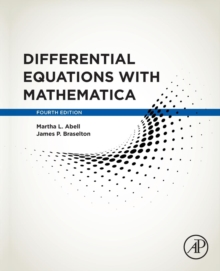# Differential Equations with Mathematica Paperback / softback

#### Description

Differential Equations with Mathematica, Fourth Edition is a supplementing reference which uses the fundamental concepts of the popular platform to solve (analytically, numerically, and/or graphically) differential equations of interest to students, instructors, and scientists. Mathematica's diversity makes it particularly well suited to performing calculations encountered when solving many ordinary and partial differential equations.

In some cases, Mathematica's built-in functions can immediately solve a differential equation by providing an explicit, implicit, or numerical solution.

In other cases, mathematica can be used to perform the calculations encountered when solving a differential equation. Because one goal of elementary differential equations courses is to introduce students to basic methods and algorithms so that they gain proficiency in them, nearly every topic covered this book introduces basic commands, also including typical examples of their application.

A study of differential equations relies on concepts from calculus and linear algebra, so this text also includes discussions of relevant commands useful in those areas.

In many cases, seeing a solution graphically is most meaningful, so the book relies heavily on Mathematica's outstanding graphics capabilities.

#### Information

• Format: Paperback / softback
• Pages: 880 pages
• Publisher: Elsevier Science Publishing Co Inc
• Publication Date:
• Category: Differential calculus & equations
• ISBN: 9780128047767

£96.00

£71.89

Free delivery within the UK